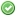rfc:static_variable_inheritance

# PHP RFC: Static variables in inherited methods

## Introduction

When a method containing static variables is inherited, the inherited method currently uses an independent set of static variables. This RFC proposes to only have one set of static variables per method.

The current behavior of static variables is illustrated in the following example:

```class A {
public static function counter() {
static \$i = 0;
return ++\$i;
}
}
class B extends A {}

var_dump(A::counter()); // int(1)
var_dump(A::counter()); // int(2)
var_dump(B::counter()); // int(1)
var_dump(B::counter()); // int(2)```

When `A::counter()` is inherited into class `B`, a separate set of static variables is used, and `A::counter()` and `B::counter()` will manage an independent counter.

This RFC proposes to instead have only one set of static variables per method, regardless of whether it is inherited:

```class A {
public static function counter() {
static \$i = 0;
return ++\$i;
}
}
class B extends A {}

var_dump(A::counter()); // int(1)
var_dump(A::counter()); // int(2)
var_dump(B::counter()); // int(3)
var_dump(B::counter()); // int(4)```

Based on a perusal of bug reports relating to static variables, this appears to be the intuitively expected behavior. Notably, it matches how static class properties already behave:

```class A {
private static \$i = 0;
public static function counter() {
return ++static::\$i;
}
}
class B extends A {}

var_dump(A::counter()); // int(1)
var_dump(A::counter()); // int(2)
var_dump(B::counter()); // int(3)
var_dump(B::counter()); // int(4)```

It also matches the behavior of other languages:

```#include <iostream>

class A {
public:
static int counter() {
static int i = 0;
return ++i;
}
};
class B : public A {
};

int main() {
std::cout << A::counter() << std::endl; // 1
std::cout << A::counter() << std::endl; // 2
std::cout << B::counter() << std::endl; // 3
std::cout << B::counter() << std::endl; // 4
return 0;
}```

A problem with the current semantics is that there is no way to preserve them when overriding the method and invoking `parent::`:

```class A {
public static function counter() {
static \$i = 0;
return ++\$i;
}
}
class B extends A {
public static function counter() {
return parent::counter();
}
}

var_dump(A::counter()); // int(1)
var_dump(A::counter()); // int(2)
var_dump(B::counter()); // int(3)
var_dump(B::counter()); // int(4)```

The general expectation is that overriding a method and calling the parent method will not change program behavior. Under the previous semantics, it does, because there is no longer a separate static variable scope associated with `B::counter()`. There is no way to make the semantics match short of copying the implementation. Under the proposed semantics, the behavior is consistent.

Finally, there are some outright bugs in the handling of static variables. For example, if static variables are declared inside a constructor (and some other magic methods), then they do end up sharing a single static variable scope:

```class A {
public function __construct() {
static \$i = 0;
var_dump(++\$i);
}
}
class B extends A {}

new A; // int(1)
new A; // int(2)
new B; // int(3)
new B; // int(4)```

In this case, the buggy behavior under current semantics, coincides with correct behavior under the proposed semantics. Of course, if this proposal is not accepted, then the behavior should be fixed for current semantics.

## Proposal

When a method containing static variables is inherited, the inherited method will share the same static variable scope:

```class A {
public static function counter() {
static \$i = 0;
return ++\$i;
}
}
class B extends A {}

var_dump(A::counter()); // int(1)
var_dump(A::counter()); // int(2)
var_dump(B::counter()); // int(3)
var_dump(B::counter()); // int(4)```

The behavior does not depend on whether the method is static or not:

```class A {
public function counter() {
static \$i = 0;
return ++\$i;
}
}
class B extends A {}

var_dump((new A)->counter()); // int(1)
var_dump((new A)->counter()); // int(2)
var_dump((new B)->counter()); // int(3)
var_dump((new B)->counter()); // int(4)```

However, static variables inside trait methods will continue to use a separate static variable scope for each use of the trait:

```trait T {
public static function counter() {
static \$i = 0;
return ++\$i;
}
}
class A {
use T;
}
class B {
use T;
}
var_dump(A::counter()); // int(1)
var_dump(A::counter()); // int(2)
var_dump(B::counter()); // int(1)
var_dump(B::counter()); // int(2)```

This is consistent with the general semantics of traits as “compiler-assisted copy and paste”. The code behaves as-if the method definition was literally pasted into both classes. This is also consistent with the behavior of static properties in traits. Additionally, it ensures that if a method is used multiple times under different aliases, they will all have distinct static variables.

## Backward Incompatible Changes

The behavior of static variables in methods changes as described above. The current behavior of static variables is not documented. I consider it to be borderline buggy. Typical memoization use-cases for static variables will not be affected (apart from memoizing more effectively).

Code that intentionally relies on the current behavior can be made compatible by indexing the static variable by the class name:

```class A {
public function counter() {
// This code works both for static and non-static methods.
static \$counters = [];
\$counters[static::class] ??= 0;
return ++\$counters[static::class];
}
}
class B extends A {
}

var_dump((new A)->counter()); // int(1)
var_dump((new A)->counter()); // int(2)
var_dump((new B)->counter()); // int(1)
var_dump((new B)->counter()); // int(2)```

This code will work the same way both before and after the proposed behavior change.

## Vote

Voting started 2021-04-14 and ended 2021-04-28.

Change static variable inheritance as proposed?
Real name Yes No
asgrim (asgrim)ashnazg (ashnazg)bwoebi (bwoebi)cmb (cmb)crell (crell)cschneid (cschneid)daverandom (daverandom)derick (derick)dharman (dharman)galvao (galvao)girgias (girgias)heiglandreas (heiglandreas)jhdxr (jhdxr)kalle (kalle)kelunik (kelunik)kguest (kguest)kinncj (kinncj)kocsismate (kocsismate)lcobucci (lcobucci)marandall (marandall)mcmic (mcmic)narf (narf)nicolasgrekas (nicolasgrekas)nikic (nikic)ocramius (ocramius)patrickallaert (patrickallaert)ramsey (ramsey)reywob (reywob)sammyk (sammyk)sebastian (sebastian)sergey (sergey)sirsnyder (sirsnyder)svpernova09 (svpernova09)tandre (tandre)theodorejb (theodorejb)trowski (trowski)twosee (twosee)yunosh (yunosh)Final result: 38 0
This poll has been closed.